black nike shoes roshe cheapnicesports.com

# Capacitors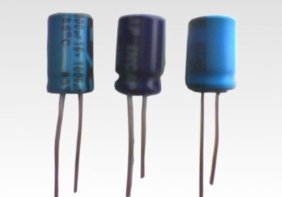Capacitor is another important component in electronics. Property to store charge makes this two pin component very special. Capacitors come in various shapes and sizes. The image above shows electrolytic capacitors. Various other types of capacitors are also available. e.g paper capacitor, ceramic capacitor, variable capacitor etc.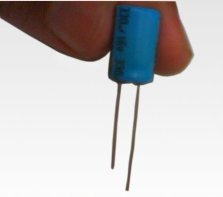Shown above is a life size capacitor. In circuit diagrams, capacitors are represented by symbols as shown below.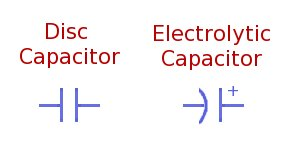The disc capacitors or non-electrolytic capacitors are polarity independent and can be used in circuits without much polarity care. Eelectrolytic capacitors are marked with -ve on them, to indicate polarity. Electrolytic capacitors should always be used in accordance with the polarity marked. Never connect +ve voltage supply to -ve pin and -ve voltage to +ve pin. Connecting electrolytic capacitor with wrong polarity may even blast the capacitor.

Capacitor can analogically be assumed as a bucket. Like amount of water a bucket can hold depends on its capacity, similarly amount of charge a capacitor can hold also depends on capacitor's capacity. This capacity is measured in "farad"(F) and generally marked on the capacitor itself e.g 10 micro-farad (µF), 100 pico-farad(pF) etc..

Along with the capacity, the capacitor's break down voltage is also marked on the capacitor e.g 12V, 16V etc.
This break down voltage is the maximum voltage can be applied to the capacitor for charging. If the charging voltage is greater than break-down voltage the capacitor may blast.

Please note that any voltage less than break-down voltage is fine, charging voltage need not be exactly same as break-down voltage. Since, only the break-down voltage is marked on the capacitor, people may think that as required charging voltage. Remember ! Voltage marked on capacitor is always break-down voltage. While purchasing capacitors take care of break-down voltage as per your circuit requirement.

Now, lets take an analogy and understand capacitor's charging behavior...

Shown below is a small beaker connected to a large tank. Lets observe a few things here.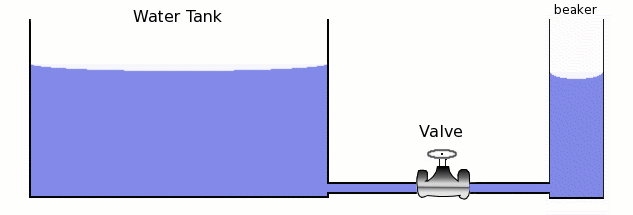1. Rate of water flow depends on the Valve.
2. Total water capacity of beaker is much less then tank.
3. Water will flow into beaker till water level in it becomes equal to that of tank.

Now, analyze the circuit shown below and observe few things here..

Probably took your legit pair and gave a fake pair to the buyer/seller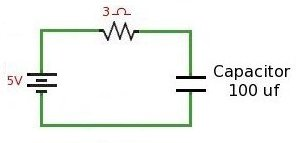1. Rate of current flow depends on the resistor.
2. Capacitor's capacity is in Farads, which is much less then battery's capacity. Hence this battery can charge number of capacitors before that really starts affecting battery's strength.
3. The current will flow in to capacitor charging it. As the capacitor charges voltage level of capacitor increases. The capacitor charges till capacitor's voltage becomes equal to battery's voltage.

Charge accumulated on capacitor after charging is given by equation...

Q = CV

Where, C is capacitance of the capacitor, V is the voltage applied for charging, and Q is charge accumulated measured in coulomb. To accumulate 1 coulomb of charge, 6.28 x 10 16 electrons are required.

When a resistor is placed in the circuit, the capacitor may take time to get fully charged. In this case time is calculated by equation ...

T = 4RC (where R is resistance, and C is capacitance)